Next: Effect of atmospheric drag Up: Secular perturbation theory Previous: Secular evolution of asteroid

# Effect of terrestrial oblateness on artificial satellite orbits

Consider a non-rotating (with respect to the distant stars) frame of reference whose origin coincides with the center of the Earth. Such a frame can be regarded as approximately inertial when we consider orbital motion in the Earth's immediate vicinity. Let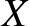,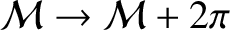,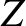be a Cartesian coordinate system in the said reference frame that is orientated such that its-axis is aligned with the Earth's (approximately) constant axis of rotation (with the terrestrial north pole lying at positive). As we saw in Section 8.9, the gravitational potential in the immediate vicinity of the Earth can be written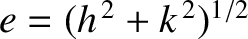(10.110)

where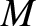is the Earth's mass,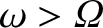its moment of inertia about the-axis, and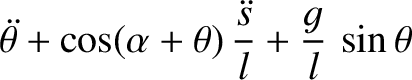its moment of inertia about an axis lying in the-plane. Here,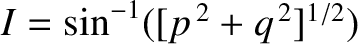and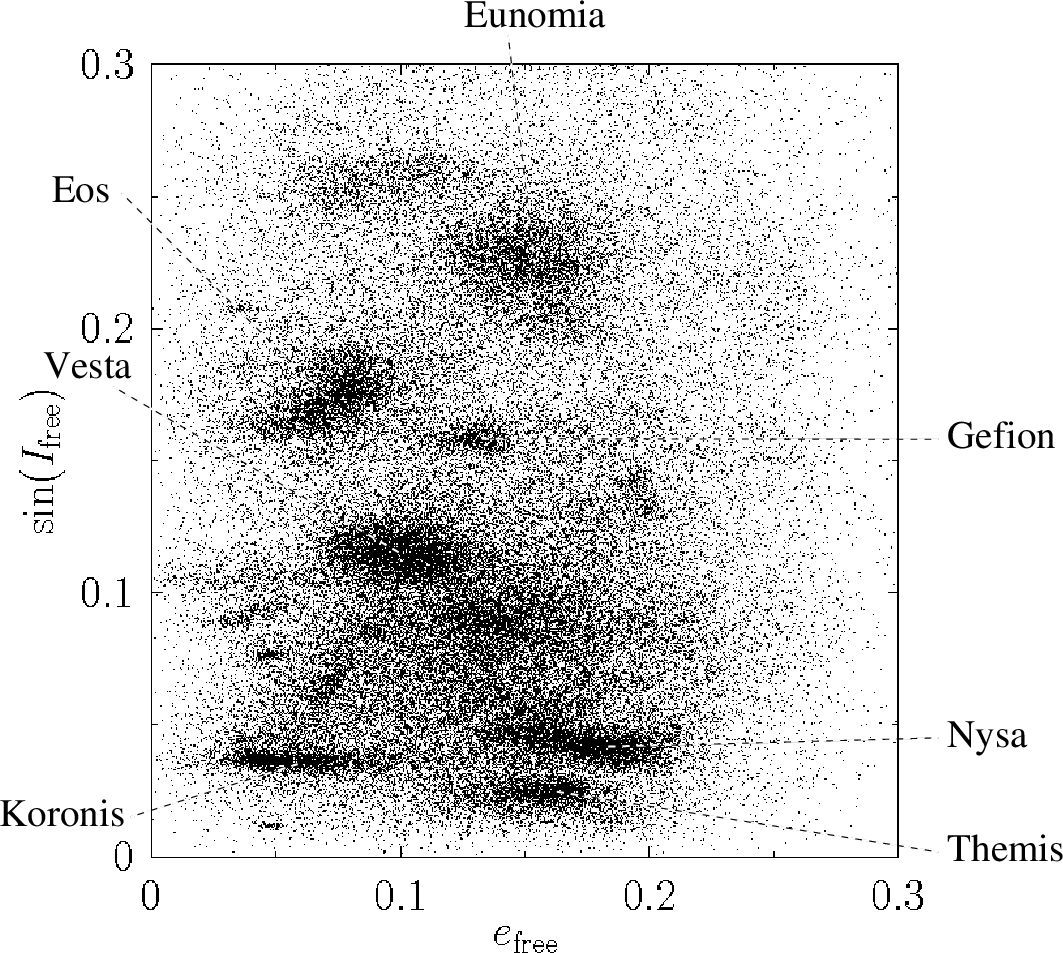are standard spherical coordinates. The first term on the right-hand side of the preceding expression is the monopole gravitational potential that would result were the Earth spherically symmetric. The second term is the small quadrupole correction to this potential generated by the Earth's slight oblateness. (See Section 6.5.) It is conventional to parameterize this correction in terms of the dimensionless quantity (Yoder 1995)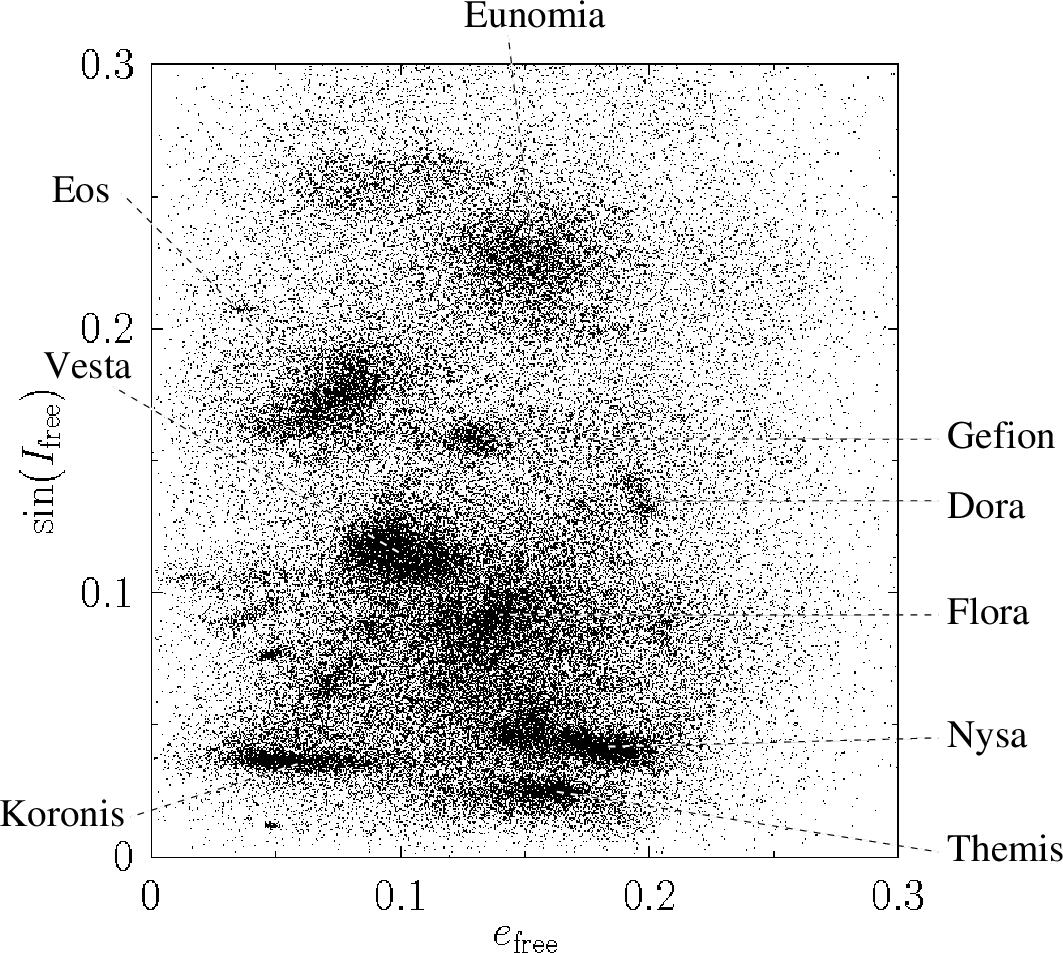(10.111)

where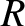is the Earth's equatorial radius. Hence, Equation (10.110) can be written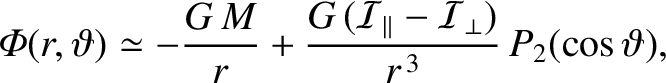(10.112)

Consider an artificial satellite in orbit around the Earth. The satellite's equation of motion in our approximately inertial geocentric reference frame takes the form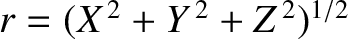(10.113)

This can be combined with Equation (10.112) to give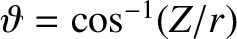(10.114)

where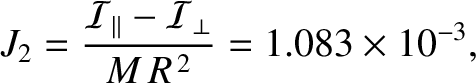, and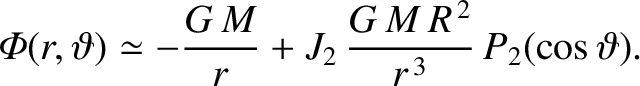(10.115)

Note that the preceding expression has exactly the same form as the canonical equation of motion, Equation (G.2), that is the starting point for orbital perturbation theory. In particular,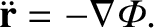is the disturbing function that describes the perturbation to the Keplerian orbit of the satellite due to the Earth's small quadrupole gravitational field.

Let the satellite's osculating orbital elements be the major radius,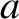; the time of perigee passage,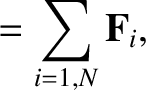; the eccentricity,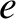; the inclination (to the Earth's equatorial plane),; the argument of the perigee,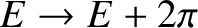; and the longitude of the ascending node (measured with respect to the vernal equinox),. (See Section 4.12.) Actually, it is more convenient to replaceby the mean anomaly,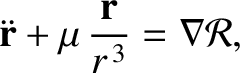, where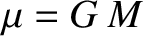is the (unperturbed) mean orbital angular velocity. According to standard orbital perturbation theory, the time evolution of the satellite's orbital elements is governed by the Lagrange planetary equations which, for the particular set of elements under consideration, take the form (see Section G.6)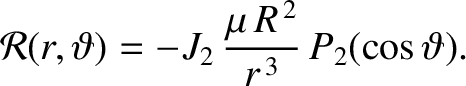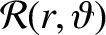(10.116)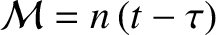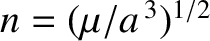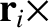(10.117)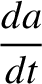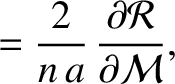(10.118)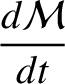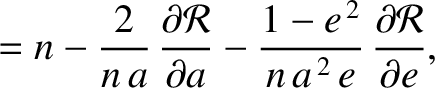(10.119)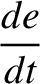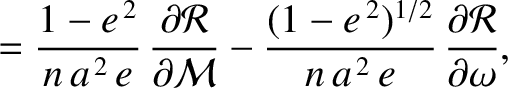(10.120) and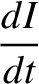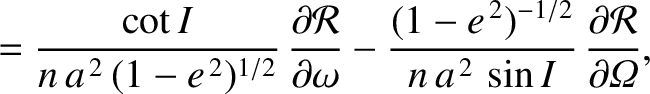(10.121)

According to Equation (4.74),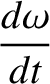(10.122)

where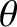is the satellite's true anomaly. Thus, expression (10.115) can be written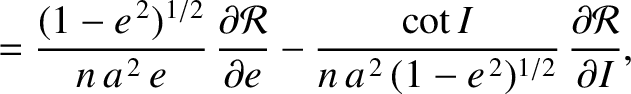(10.123)

We are primarily interested in the secular evolution of the satellite's orbital elements; that is, the evolution on timescales much longer than the orbital period. We can concentrate on this evolution, and filter out any relatively short-term oscillations in the elements, by averaging the disturbing function over an orbital period. In other words, in Equations (10.116)-(10.121), we need to replace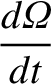by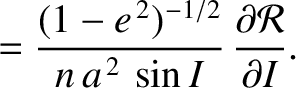(10.124)

where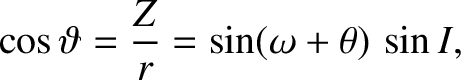. Here, we have made use of the fact thatis a constant of the motion in a Keplerian orbit. (See Chapter 4.) A Keplerian orbit is also characterized by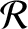. Hence, the previous two equations can be combined to give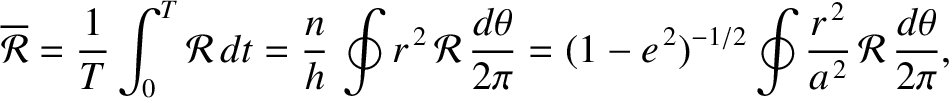(10.125)

which evaluates to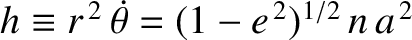(10.126)

Substitution of this expression into Equations (10.116), (10.118), and (10.119) (recalling that we are replacingby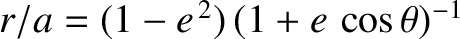) reveals that there is no secular evolution of the satellite's orbital major radius,, eccentricity,, and inclination,, due to the Earth's oblateness (becausedoes not depend on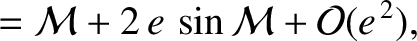,, or). On the other hand, according to Equations (10.120) and (10.121), the oblateness causes the satellite's perigee and ascending node to precess at the constant rates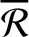(10.127)

and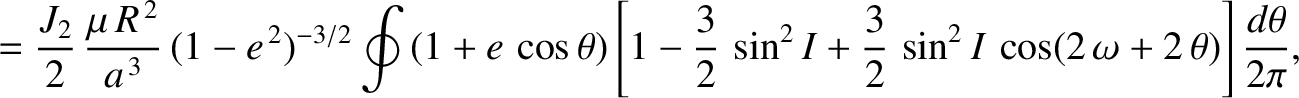(10.128)

respectively. These formulae suggest that the precession of the ascending node is always in the opposite sense to the orbital motion; that is, it is retrograde. Note, however, that the ascending node remains fixed in the special case of a so-called polar orbit that passes over the terrestrial poles (i.e.,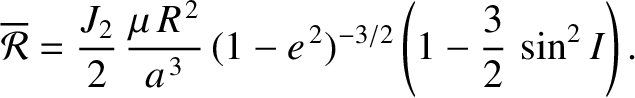). The formulae also suggest that the perigee precesses in a prograde fashion when, in a retrograde fashion when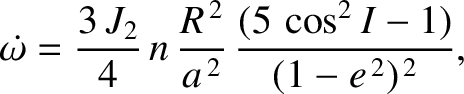, and remains fixed when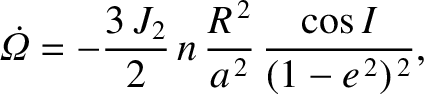. In other words, the perigee of an orbit lying in the Earth's equatorial plane precesses in the same direction as the orbital motion, the perigee of a polar orbit precesses in the opposite direction, and the perigee of an orbit that is inclined at the critical angle of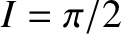to the Earth's equatorial plane does not precess at all. Finally, substitution of expression (10.126) into Equation (10.117) reveals that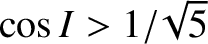(10.129)

We conclude that the Earth's oblateness causes the mean angular velocity of an orbiting satellite (which, by definition, is equal to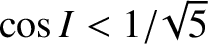) to slightly exceed the Keplerian valuefor low inclination orbits that satisfy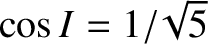, to be slightly less than the Keplerian value for high inclination orbits such that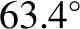, and to exactly equal the Keplerian value when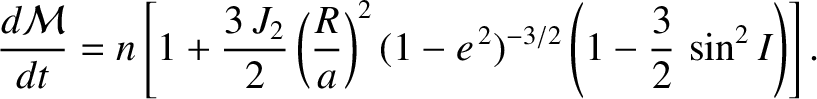.

It should be noted that the dimensionless quadrupole moment of the Earth's gravitational field,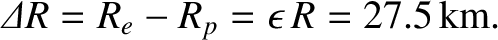, (as well as higher-order coefficients such as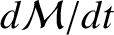; see Exercise 3) is most accurately determined via observations of the precession rates of the perigees and ascending nodes of orbiting satellites.Next: Effect of atmospheric drag Up: Secular perturbation theory Previous: Secular evolution of asteroid
Richard Fitzpatrick 2016-03-31